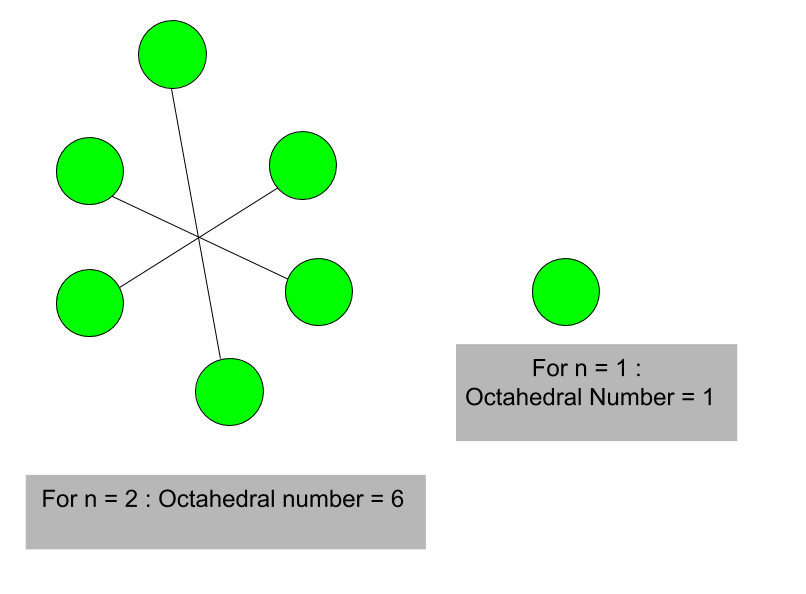# Octahedral Number

Given a number n, the task is to find n-th octahedral number.
An octahedral number belongs to a figurate number and it is the number of spheres in an octahedron built from closely packed spheres. First, a few octahedral numbers (where n = 0, 1, 2, 3…….) are 0, 1, 6, 19, and so on.

Examples :

Input:
Output: 44

Input:
Output: 344Formula for nth octahedral number:

`n * (2n2+1) / 3`

## C++

 `// C++ program to find nth` `// octahedral number` `#include ` `using` `namespace` `std;`   `// Function to find` `// octahedral number` `int` `octahedral_num(``int` `n)` `{` `    ``// Formula to calculate nth` `    ``// octahedral number` `    ``return` `n * (2 * n * n + 1) / 3;` `}`   `// Drivers code` `int` `main()` `{` `    ``int` `n = 5;`   `    ``// print result` `    ``cout << n << ``"th Octahedral number: "``;` `    ``cout << octahedral_num(n);` `    ``return` `0;` `}`

## Java

 `// Java program to find nth octahedral` `// number` `import` `java.io.*;`   `class` `GFG {`   `    ``// Function to find octahedral number` `    ``static` `int` `octahedral_num(``int` `n)` `    ``{`   `        ``// Formula to calculate nth` `        ``// octahedral number` `        ``// and return it into main function.` `        ``return` `n * (``2` `* n * n + ``1``) / ``3``;` `    ``}`   `    ``// Driver Code` `    ``public` `static` `void` `main(String[] args)` `    ``{` `        ``int` `n = ``5``;` `        ``// print result` `        ``System.out.print(n + ``"th Octahedral"` `                         ``+ ``" number: "``);` `        ``System.out.println(octahedral_num(n));` `    ``}` `}`

## Python3

 `# Python 3 program to find nth` `# octahedral number`   `# Function to find` `# octahedral number` `def` `octahedral_num(n) :` `    `  `    ``# Formula to calculate nth` `    ``# octahedral number` `    ``return` `n ``*` `(``2` `*` `n ``*` `n ``+` `1``) ``/``/` `3`   `# Driver Code` `if` `__name__ ``=``=` `'__main__'` `:` `        `  `    ``n ``=` `5` `    ``print``(n,``"th Octahedral number: "` `                ``, octahedral_num(n))`   `# This code is contributed ajit.`

## C#

 `// C# program to find nth ` `// Octahedral number` `using` `System;`   `class` `GFG` `{` `    `  `    ``// Function to find ` `    ``// octahedral number` `    ``static` `int` `octahedral_num(``int` `n)` `    ``{`   `        ``// Formula to calculate ` `        ``// nth octahedral number` `        ``// and return it into ` `        ``// main function.` `        ``return` `n * (2 * n * ` `                    ``n + 1) / 3;` `    ``}`   `    ``// Driver Code` `    ``static` `public` `void` `Main ()` `    ``{` `        ``int` `n = 5;` `        `  `        ``// print result` `        ``Console.Write(n + ``"th Octahedral"` `                        ``+ ``" number: "``);` `        ``Console.WriteLine(octahedral_num(n));` `    ``}` `}`   `// This code is Contributed by m_kit`

## PHP

 ` `

## Javascript

 ``

Output

`5th Octahedral number: 85`

Time Complexity: O(1) because constant operations are being performed
Auxiliary Space: O(1)

Feeling lost in the world of random DSA topics, wasting time without progress? It's time for a change! Join our DSA course, where we'll guide you on an exciting journey to master DSA efficiently and on schedule.
Ready to dive in? Explore our Free Demo Content and join our DSA course, trusted by over 100,000 geeks!

Previous
Next